Courses

# Test: Thermodynamics 1 - From Past 28 Years Questions

## 36 Questions MCQ Test Chemistry 28 Years Past year papers for NEET/AIPMT Class 11 | Test: Thermodynamics 1 - From Past 28 Years Questions

Description
This mock test of Test: Thermodynamics 1 - From Past 28 Years Questions for NEET helps you for every NEET entrance exam. This contains 36 Multiple Choice Questions for NEET Test: Thermodynamics 1 - From Past 28 Years Questions (mcq) to study with solutions a complete question bank. The solved questions answers in this Test: Thermodynamics 1 - From Past 28 Years Questions quiz give you a good mix of easy questions and tough questions. NEET students definitely take this Test: Thermodynamics 1 - From Past 28 Years Questions exercise for a better result in the exam. You can find other Test: Thermodynamics 1 - From Past 28 Years Questions extra questions, long questions & short questions for NEET on EduRev as well by searching above.
QUESTION: 1

### What is the entropy change (in JK–1 mol–1) when one mole of ice is converted into water at 0º C? (The enthalpy change for the conversion of ice to liquid water is 6.0 kJ mol–1 at 0ºC) 

Solution: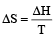ΔS (per mole )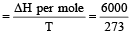= 21.98 JK -1mol-1

QUESTION: 2

### The densities of graphite and diamond at 298 K are 2.25 and 3.31g cm–3, respectively. If the standard free energy difference (ΔGº) is equal to 1895 J mol–1, the pressure at which graphite will be transformed into diamond at 298 K is: 

Solution:

ΔG = – PΔV = Work done
ΔV is the change in molar volume in the conversion of graphite to diamond.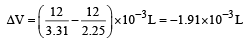Work done = – (–1.91 × 10–3) × P × 101.3 J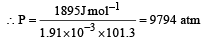∵ 1 atm = 105 × 1.013 Pa
⇒ P = 9.92 x 108 Pa

QUESTION: 3

### For which one of the following equations is ΔHºreact equal to ΔHfº for the product? 

Solution:

► The heat of formation is defined as the heat generated or observed when the compound is formed from its component elements in their standard state.

• By the definition (C) and (A) are incorrect as the starting material are compounds.
• (B) is incorrect as ozone is not the standard state for oxygen.
• (D) is correct as it satisfies the definition of the heat of formation.
QUESTION: 4

For the reaction C3H8(g) + 5O2(g) → 3CO2(g) + 4H2O(l) at constant temperature, ΔH – ΔE is: 

Solution:

ΔH = ΔE + nRT
Δng = 3 - (1 + 5) = -3
ΔH - ΔE = (-3 RT)

QUESTION: 5

If the bond energies of H-H, Br-Br, and HBr are 433, 192 and 364 kJ mol–1 respectively, the ΔH° for the reaction H2(g) + Br2(g) → 2HBr(g) is: 

Solution:

H2(g) + Br2(g) → 2HBr(g)

ΔHº = (B.E.)react - (B.E.)prod
⇒ ΔHº = (433 + 192) - (2 x 364) = 625 – 728 = – 103 kJ

QUESTION: 6

The standard enthalpy and standard entropy changes for the oxidation of ammonia at 298 K are -382.64 kJ mol–1 and -145.6 JK–1 mol–1, respectively.
Standard Gibb's energy change for the same reaction at 298 K is: 

Solution:

ΔG = ΔH - TΔS
ΔG = -382.64 - (298 x 145.6 x 10-3) = -339.3 kJ mol-1

QUESTION: 7

Considering entropy(S) as a thermodynamic parameter, the criterion for the spontaneity of any process is: 

Solution:

For a spontaneous process, ΔStotal is always positive.

QUESTION: 8

The work done during the expansion of a gas from a volume of 4 dm3 to 6 dm3 against a constant external pressure of 3 atm is: (1L atm  = 101.32 J) 

Solution:

W = – pΔV
W = -3(6 - 4) = - 6 L atm = - 6 x 101.32 J = (approx) - 608 J

QUESTION: 9

The absolute enthalpy of neutralisation of the reaction: MgO (s) + 2HCl (aq) —→ MgCl2(aq) + H2O (l) will be:

Solution:

As MgO is a oxide of  weak base hence  some energy is lost to break MgO (s). Hence enthalpy is less than –57.33 kJ mol–1.

QUESTION: 10

A reaction occurs spontaneously if: 

Solution:

For a spontaneous reaction, ΔG  is always –ve.
Since, ΔG = ΔH – TΔS
Therefore, ΔS = +ve, ΔH = +ve and  TΔS > ΔH.

QUESTION: 11

Which of the following pairs of a chemical reaction is certain to result in a spontaneous reaction? 

Solution:

The measure of the disorder of a system is nothing but Entropy.
For a spontaneous reaction, ΔG < 0. As per Gibbs Helmnoltz equation, ΔG = ΔH – TΔS.
Thus, ΔG is –ve, if ΔH = –ve (exothermic) and ΔS = +ve (increasing disorder).

QUESTION: 12

The enthalpy of hydrogenation of cyclohexene is –119.5 kJ mol–1. If the resonance energy of benzene is –150.4 kJ mol–1, its enthalpy of hydrogenation would be: 

Solution: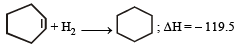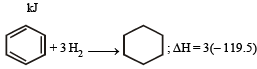= – 348.5 kJ

The resonance energy provides extra stability to the benzene molecule so it has to be overcome for hydrogenation to take place.
So, ΔH = – 358.5 – (–150.4) = –208.1 kJ

QUESTION: 13

The enthalpy and entropy change for the reaction Br2(l) + Cl2(g) → 2BrCl(g) are 30kJ mol–1 and 105 JK–1 mol–1 respectively.The temperature at which the reaction will be in equilibrium is: 

Solution:

We know that, ΔG = ΔH – TΔS
When the reaction is in equilibrium, ΔG = 0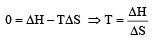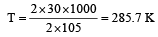QUESTION: 14

Identify the correct statement for change of Gibbs energy for a system (ΔGsystem) at constant temperature and pressure: 

Solution:
• If ΔGsystem = 0 the system has attained equilibrium is correct.
• (d) is confusing as to when ΔG > 0, the process may be spontaneous when it is coupled with a reaction which has ΔG < 0 and total ΔG is negative.
QUESTION: 15

Assume each reaction is carried out in an open container. For which reaction will ΔH = ΔE? 

Solution:

We know that: ΔH = ΔE + PΔV
In the reactions, H2 + Br2 → 2HBr there is no change in volume or ΔV = 0.
So, ΔH = ΔE for this reaction.

QUESTION: 16

Consider the following reactions: 

(i)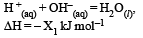(ii)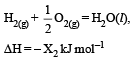(iii)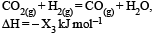(iv)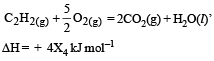Enthalpy of formation of H2O (l) is

Solution:

Reaction (ii) shows the formation of H2O, and the X2 represents the enthalpy of formation of H2O because as the definition suggests that the enthalpy of formation is the heat evolved or absorbed when one mole of a substance is formed from its constituent atoms.

QUESTION: 17

Given that bond energy of H–H and Cl–Cl is 430 kJ mol–1 and 240 kJ mol–1 respectively and ΔHf for HCl is - 90 kJ mol–1, bond enthalpy of HCl is: 

Solution: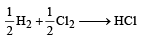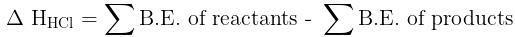-90 = [1/2 x 430 + 1/2 x 240] - B.E. of HCl
∴  B.E. of HCl = 215 + 120 + 90 = 425 kJ mol–1

QUESTION: 18

Which of the following are not state functions? 
(I) q + w
(II) q
(III) w
(IV) H - TS

Solution:

► A property whose value doesn’t depend on the path taken to reach that specific value is known as state functions or point functions.
► In contrast, those functions which do depend on the path from two points are known as path functions.

Heat (q) and Work (w) are not state functions but (q + w) is a state functions.
H – TS (i.e. G) is also a state function.

Thus, II and III are not state functions so the correct answer is option (d).

QUESTION: 19

For the gas phase reaction, which of the following conditions is correct? 
PCl5(g) ⇌ PCl3​(g) + Cl2​(g)

Solution: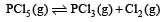The reaction given is an example of a decomposition reaction
Since, decomposition reactions are endothermic in nature, therefore, ΔH > 0.
Δn = (1+1) – 1= +1
Hence more molecules are present in products which shows more randomness i.e. ΔS > 0 (ΔS is positive).

QUESTION: 20

Bond dissociation enthalpy of H2, Cl2, and HCl are 434, 242, and 431 kJ mol–1 respectively. Enthalpy of formation of HCl is: 

Solution:

The reaction of formation of HCl: H2 + Cl2 → 2HCI
⇒ Enthalpy of formation of 2HCl = - (862 - 676) = -186 kJ.
∴ Enthalpy of formation of HCl = -(186/2) kJ = -93 kJ

QUESTION: 21

The values of ΔH and ΔS for the reaction, C(graphite) + CO2 (g) → 2CO(g) are 170 kJ and 170 JK–1, respectively. This reaction will be spontaneous at: 

Solution:

ΔG = ΔH – T Δ S
At equilibrium, ΔG = 0
⇒ 0 = (170 × 103 J) – T (170 JK– 1)
⇒ T = 1000 K
For spontaneity, ΔG is – ve, which is possible only if T > 1000 K.

QUESTION: 22

From the following bond energies: 
H – H bond energy: 431.37 kJ mol–1
C = C bond energy: 606.10 kJ mol–1
C – C bond energy: 336.49 kJ mol–1
C – H bond energy: 410.50 kJ mol–1

Enthalpy for the reaction, will be: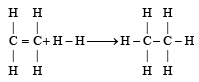Solution:

Enthalpy of reaction = B.E(Reactant)– B.E(Product)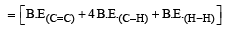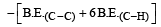ΔHreaction = [606.1 + (4 × 410.5) + 431.37)] –  [336.49 + (6 × 410.5)]  = –120.0 kJ mol–1

QUESTION: 23

Standard entropies of X2 , Y2 and XY3 are 60, 40 and 50 JK–1mol–1 respectively. For the reaction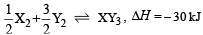to be at equilibrium, the temperature should be: 

Solution:

ΔS for the reaction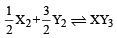ΔS = 50 – (30 + 60) = – 40 J
For equilibrium ΔG = 0 = ΔH – TΔS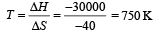QUESTION: 24

For vapor ization of water at 1 atmospheric pressure, the values of ΔH and ΔS are 40.63 kJmol–1 and 108.8 JK–1 mol–1, respectively. The temperature when Gibbs energy change (ΔG) for this transformation will be zero, is: 

Solution: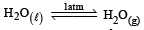ΔH = 40630J mol –1
ΔS = 108.8JK–1 mol –1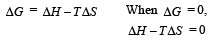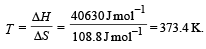∴ Correct choice : (d)

QUESTION: 25

Match List -I (Equations) with List-II (Type of processes) and select the correct option.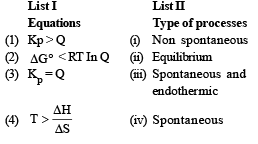Options: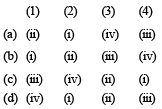Solution: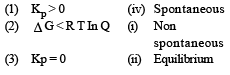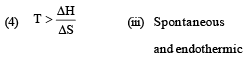∴ Correct choice : (d)

QUESTION: 26

The following two reactions are known :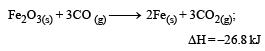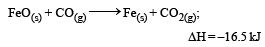The value of ΔH for the following reaction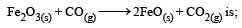Solution: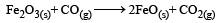ΔH = –26.8 + 33.0 = + 6.2 kJ

∴   Correct choice : (a)

QUESTION: 27

If the enthalpy change for the transition of liquid water to steam is 30 kJ mol–1 at 27ºC, the entropy change for the process would be: 

Solution:

Given ΔH = 30 kJ mol–1 T = 273 + 27 = 300 K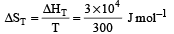= 100J mol–1 K–1

QUESTION: 28

Enthalpy change for the reaction, 4H(g) → 2H2 (g)  is - 869.6 kJ. The dissociation energy of H–H bond is: 

Solution:

Given:
4H(g) → 2H2 (g); ΔH = - 869.6 kJ
i.e. 2H2 (g) → 4H(g); ΔH = 869.6 kJ

⇒ H2 (g) → 2H(g); ΔH = (869.6) * 1/2 = 434.8 kJ

QUESTION: 29

Consider the following processes :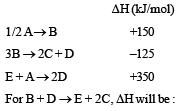[2011M]

Solution: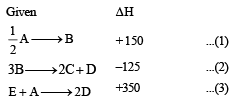To calculate ΔH operate 2 × eq. (1) + eq. (2) – eq. (3)
ΔH = 300 – 125 – 350 = – 175KJ/mol

QUESTION: 30

In which of the following reactions, standard entropy change (ΔS°) is positive and standard Gibb’s energy change (ΔG°) decreases sharply with increasing temperature? 

Solution:

Since in the first reaction gaseous products are forming from solid carbon hence entropy will increase i.e. ΔSº = +ve.

Since, ΔG° = ΔH° – TΔS
Hence the value of ΔG decrease on increasing temperature.

QUESTION: 31

The enthalpy of fusion of water is 1.435 kCal/mol.The molar entropy change for the melting of ice at 0°C is: 

Solution: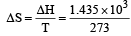= 5.260 cal / mol-K

QUESTION: 32

Standard enthalpy of vapourisation Δvap H°  for water at 100°C is 40.66 kJ mol–1. The internal energy of vaporisation of water at 100°C (in kJ mol–1) is : 

(Assume water vapour to behave like an ideal gas).

Solution:

vapH° = 40.66 kJ mol-1
H2O(l) ⇋ H2O(g)
∆ng = 1-0 = 1
∆H = ∆U + ∆ngRT
∆U = ∆H-∆ngRT
= 40,660 - 8.314×373
= 37558.878 J mol-1 or 37.56kJ mol-1

QUESTION: 33

Equal volumes of two monoatomic gases, A and B, at the same temperature and pressure are mixed. The ratio of specific heats (Cp/Cv) of the mixture will be: [2012 M]

Solution: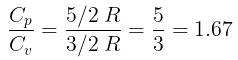QUESTION: 34

The Gibbs’ energy for the decomposition of Al2O3 at 500°C is as follows :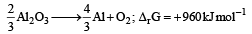The potential difference needed for the electrolytic reduction of aluminium oxide (Al2O3) at 500°C is at least : [2012 M]

Solution:

ΔG = -nFE°

for the reaction n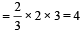960 × 103 = – 4 × 96500 × E°
E° = – 2.5 volt
So, it needed 2.5 volt for reduction

QUESTION: 35

When 5 liters of a gas mixture of methane and propane is perfectly combusted at 0°C and 1 atmosphere, 16 liters of oxygen at the same temperature and pressure is consumed. The amount of heat released from this combustion in kJ is: [NEET Kar. 2013]
ΔHcomb (CH4) = 890 kJ mol–1
ΔHcomb (C3H8) = 2220 kJ mol–1

Solution: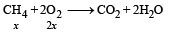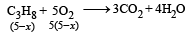2x + 5(5– x) = 16
⇒  x = 3L
∴  Heat released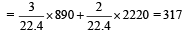QUESTION: 36

Three thermochemical equations are given below:

(i)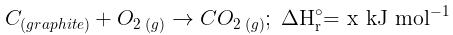(ii)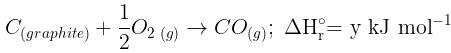(iii)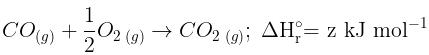Based on the above equations, find out which of the relationship given below is correct? [NEET Kar. 2013]

Solution:

Applying Hess’s law, equation (i) can be obtained by adding equations (ii) and (iii).
∴ x = y + z